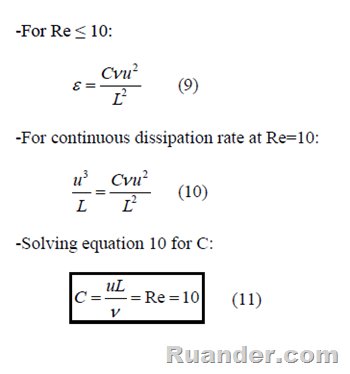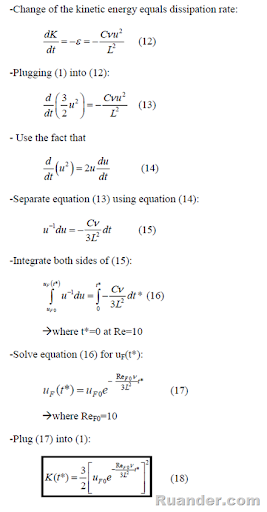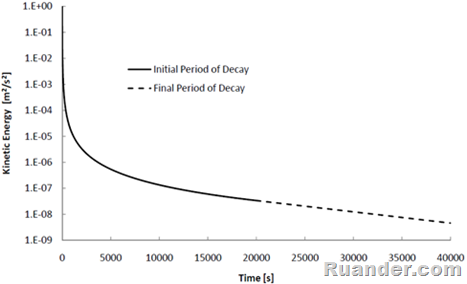## Pages

### Decay of Isotropic Turbulence in a Box

A classical example problem in turbulence is that of decay of isotropic turbulence in a box. This problem was proposed by Tennekes and Lumley  at the end of chapter 1 and it reads as follow:I have solved this problem at least twice while studying turbulence and I am sure that many of you have also solved or will solve this problem. Therefore, I decided to post my solution to the problem. For the derivation of an expression for the decay of the kinetic energy as a function of time using the inviscid estimate we have the following:For Reynolds numbers (Re) less than 10, a viscous dissipation term is used. The value of C in the suggested expression can be found by enforcing the dissipation rate to be continuous at Re=10 :To derive an expression for the decay of the kinetic energy during the final period of decay, Re<10, we can use the same methodology as used for the initial period of decay, Re>10.One can use equation (7) to find out the time it takes to enter the final period of decay. The result comes out to be about 19,997 s or about 5.55 hr for the values given (this is also the time at which t*=0).  The following semi-log plot shows the decay of turbulent kinetic energy as a function of time as predicted by equation (8) and (18) using the values provided.The solution of this problem assumes that the wall effects are negligible. At high Re this assumption is correct since the viscous dissipation is much smaller than turbulent dissipation. However, as the Re decreases, the effect of viscous dissipation at the wall becomes important and this is why we are including a final period of decay which includes the effect of the fluid viscosity.

References:

Tennekes, H., Lumley, J., L., 1972, A First Course in Turbulence, MIT Press